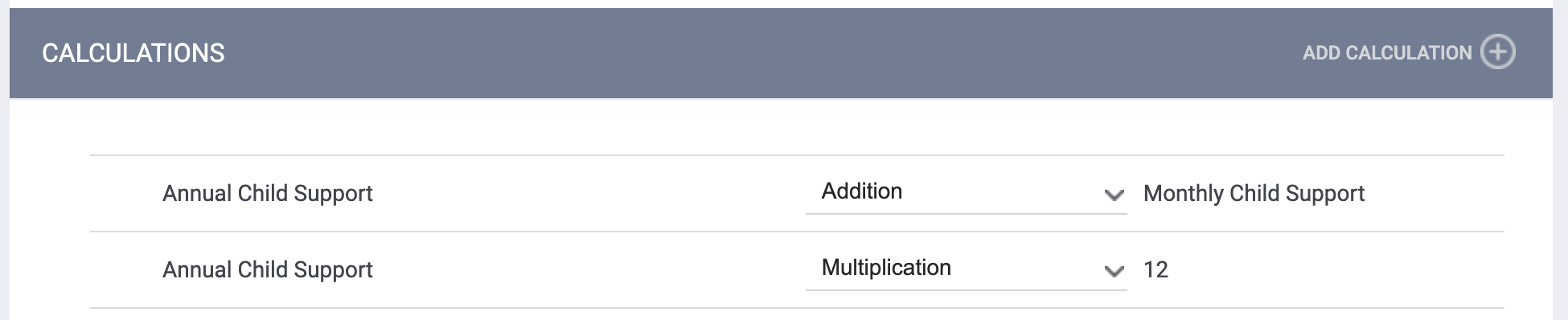# Screen Field Editor: Calculations

Calculations are used to create a response for the field based on mathematical formulas applied to other fields in the screen. Any field with a Field Type of "Number" or "Dollar" can have a calculation added to it and be used in a calculation.

For example, suppose we have a field for entering the amount of money in a client's savings account (we'll call it Savings), and a field for entering the amount of money in a client's checking account (we'll call it Checking), and we want to calculate the client's total available balance by adding the value for Savings to the value for Checking.

First, we ensure that the fields we will need for this calculation have been created and added to the SCREEN EDITOR for the screen where we want to use the calculation. In this example, we need the Savings field, the Checking field, and a field for calculating and displaying the total number (we'll call it Total Available).Next, we need to add individual steps to build the desired mathematical equation from a starting value of 0. To do that, we click the Edit icon next to the field where we want the calculation result to appear (in this case, Total Available).The SCREEN FIELD EDITOR opens. Scroll down to the CALCULATIONS section.

Note: if you do not see a CALCULATIONS section, check to make sure that you selected a "Number" or "Dollar" field to edit.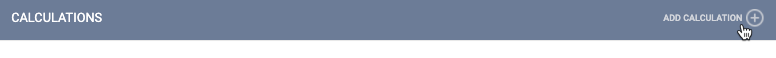Click ADD CALCULATION. The ADD CALCULATION pop-up appears. This pop-up allows you to apply one mathematical operation to one value.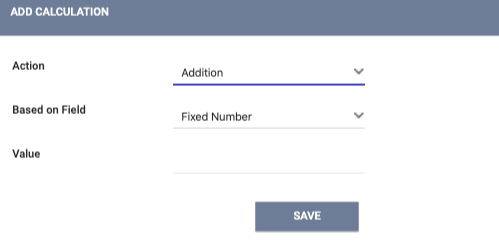Action: this picklist allows you to select the desired mathematical operation (Addition, Subtraction, Multiplication, or Division).

Based on Field: this picklist allows you to select the field or the fixed number to which the mathematical operation will be applied. When you click the drop-down arrow, you will see the "Fixed Number" option displayed, along with the fields on the screen that have a Field Type of "Number" or "Dollar." If the screen you are editing does not contain any other "Number" or "Dollar" fields, the only option available here will be "Fixed Number."

Value: the value for the fixed number to which the Action will be applied. It only applies if you choose "Fixed Number" from the Based on Field picklist. If you select a field from the Based on Field picklist, Value will be hidden.

Select the Action, the Based on Field, and the Value (if applicable), then click SAVE.

Repeat the ADD CALCULATION process as many times as needed to construct the desired equation.

Returning to our example, we'll need to do the ADD CALCULATION process two times: once to add Savings, and once to add Checking.

So we will start by clicking ADD CALCULATION, selecting "Addition" for the Action, selecting "Savings" as the Based on Field, then clicking SAVE.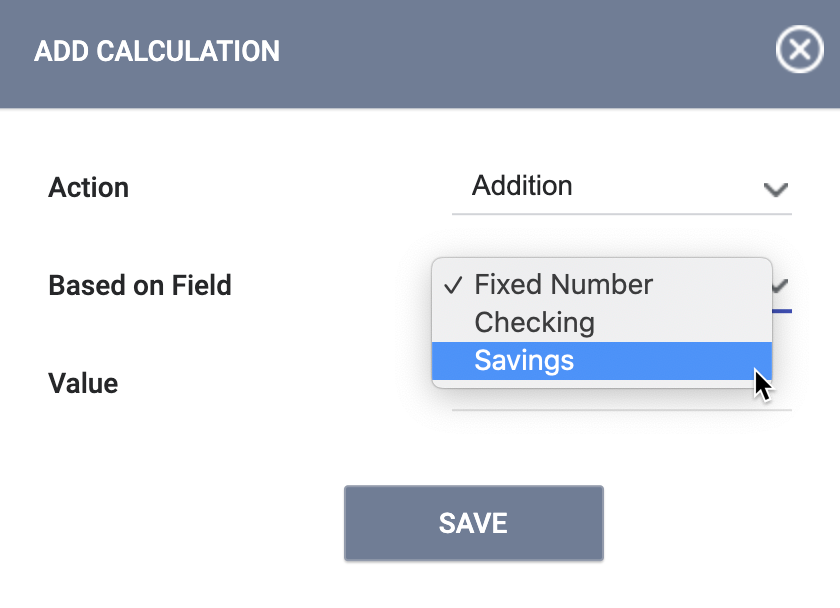Savings has now been added to the equation:

Total Available = 0 + Savings

Next, we need to add Checking to the equation. So we click ADD CALCULATION again and select "Addition" again, but this time we choose "Checking" as the Based on Field. Click SAVE.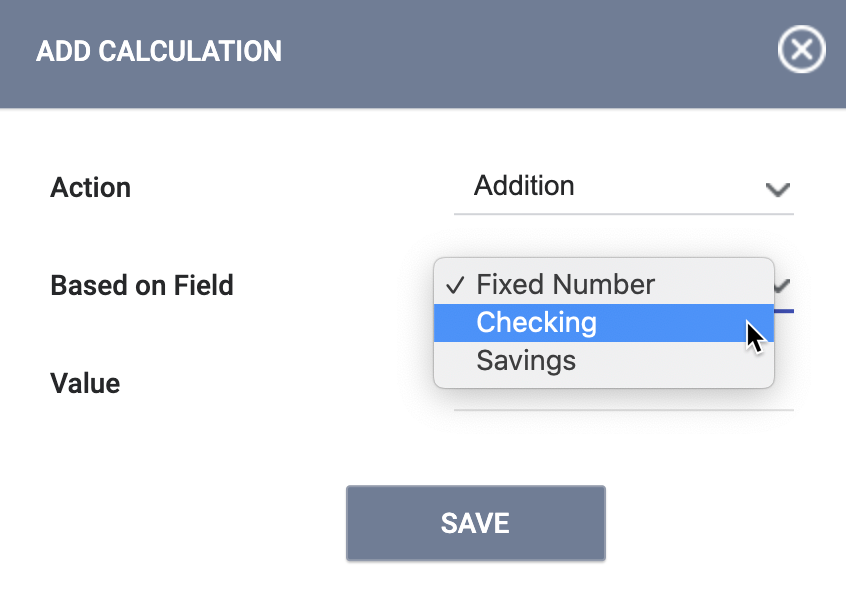Checking has now been added to the equation:

Total Available = 0 + Savings + Checking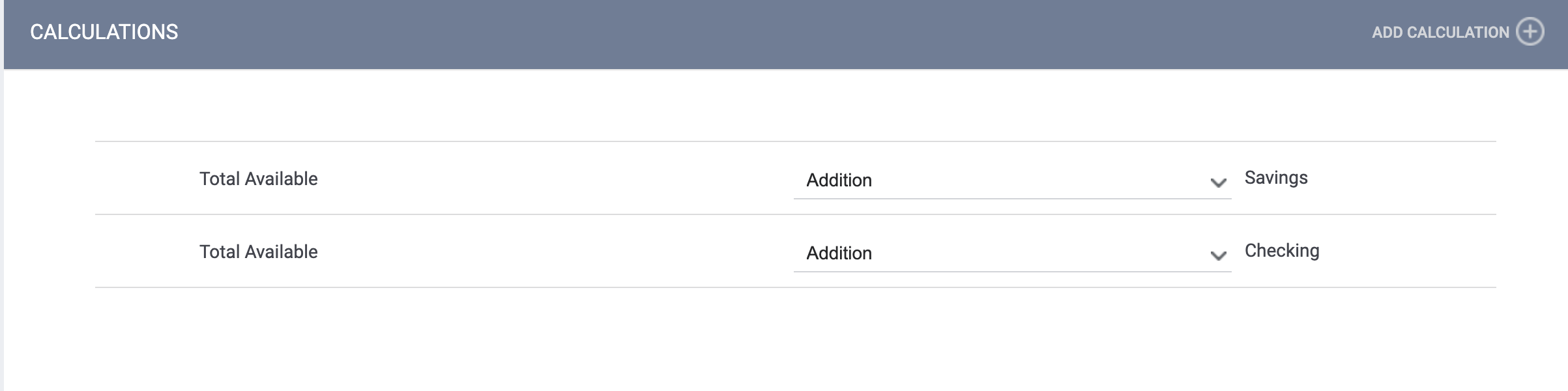When we go back to the SCREEN EDITOR for this screen, we see that our Total Available field is displayed with a calculator icon to indicate that it includes a calculation.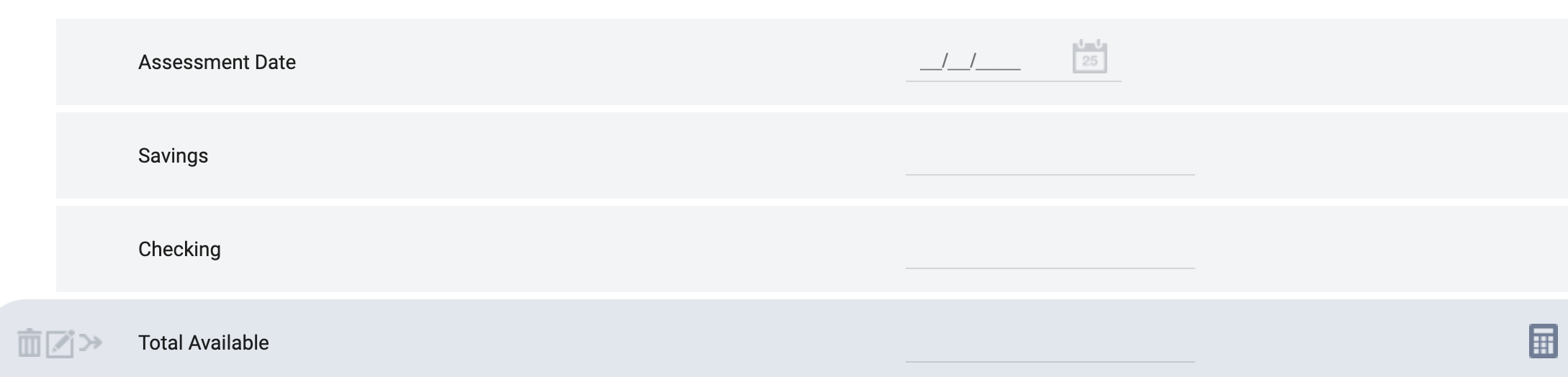To complete our example, let's look at what happens when this screen is in use. As shown below, the Total Available field starts at 0 and is updated with the new total as you enter Savings and CheckingNote: If you want to start with a multiplication or division step, keep in mind that the initial value for the equation is 0. Before you can multiply or divide a value, you will first need to add that value to the equation (otherwise, you will just be multiplying or dividing by 0). For example, if you want to calculate an Annual Child Support amount by multiplying Monthly Child Support by 12:

Step 1: Add Monthly Child Support to the equation.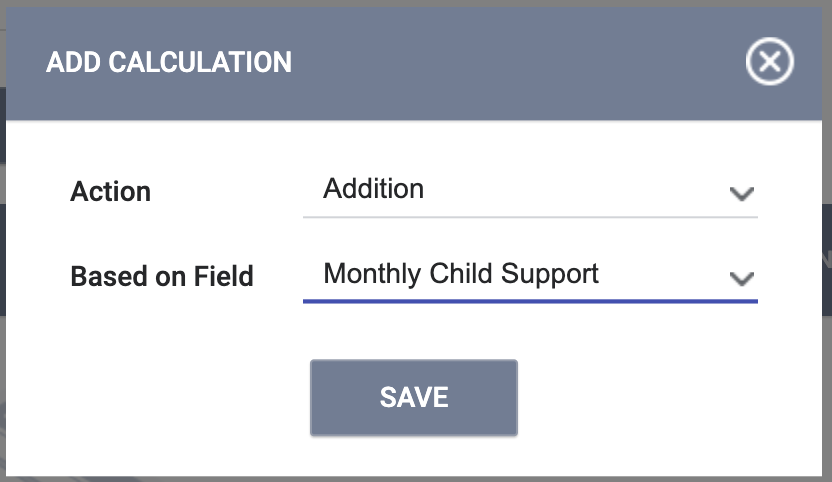Step 2: Multiply by 12.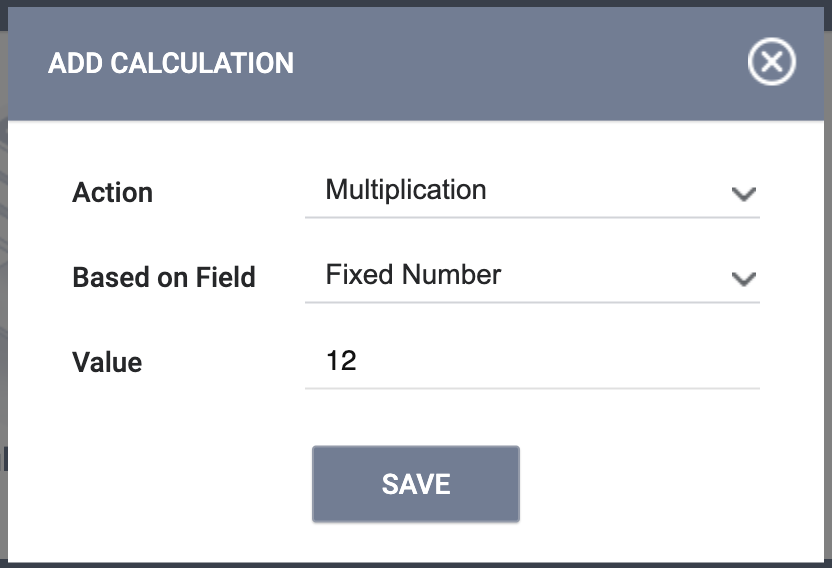The resulting calculation is Annual Child Support = 0 + Monthly Child Support x 12.